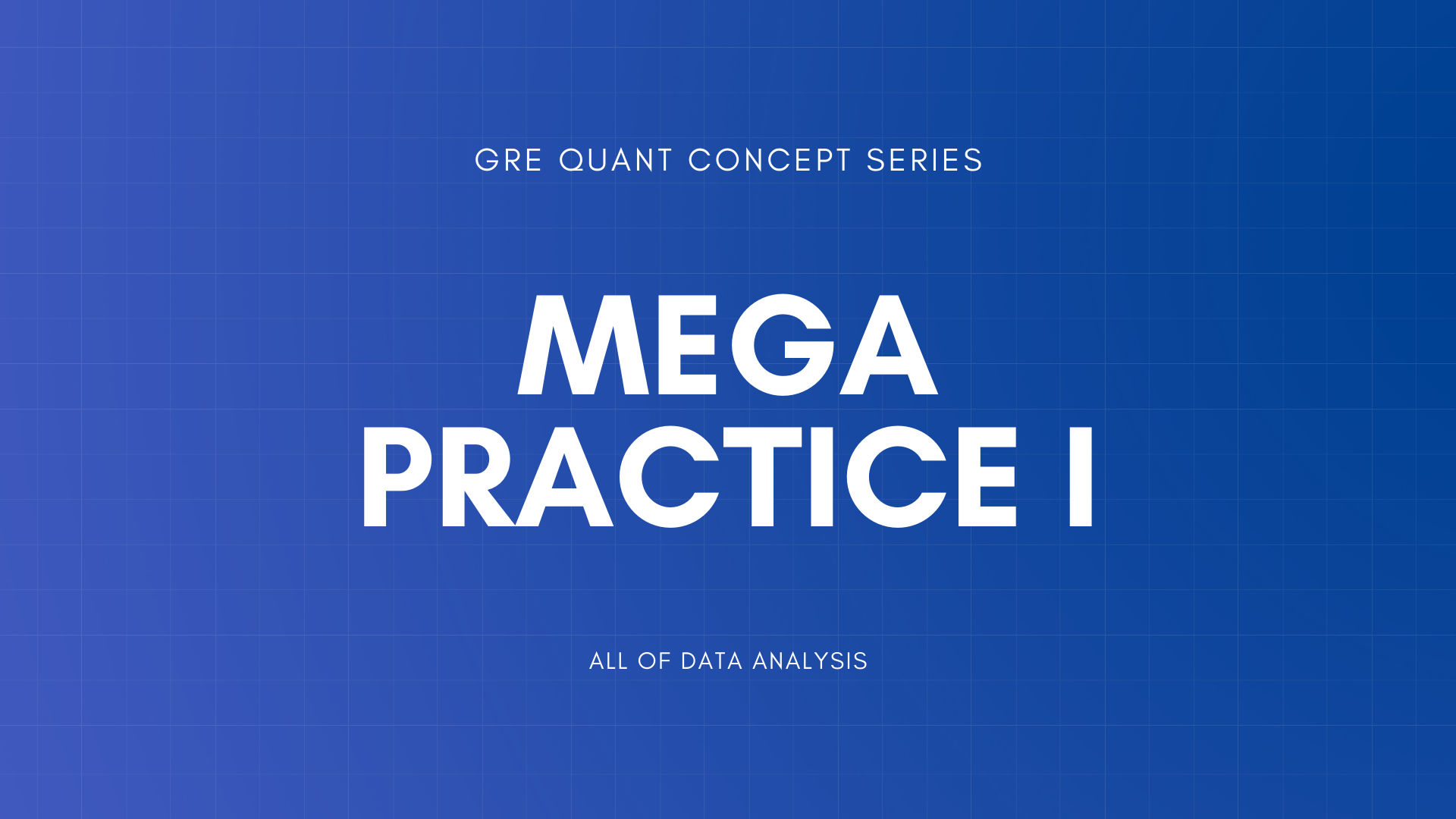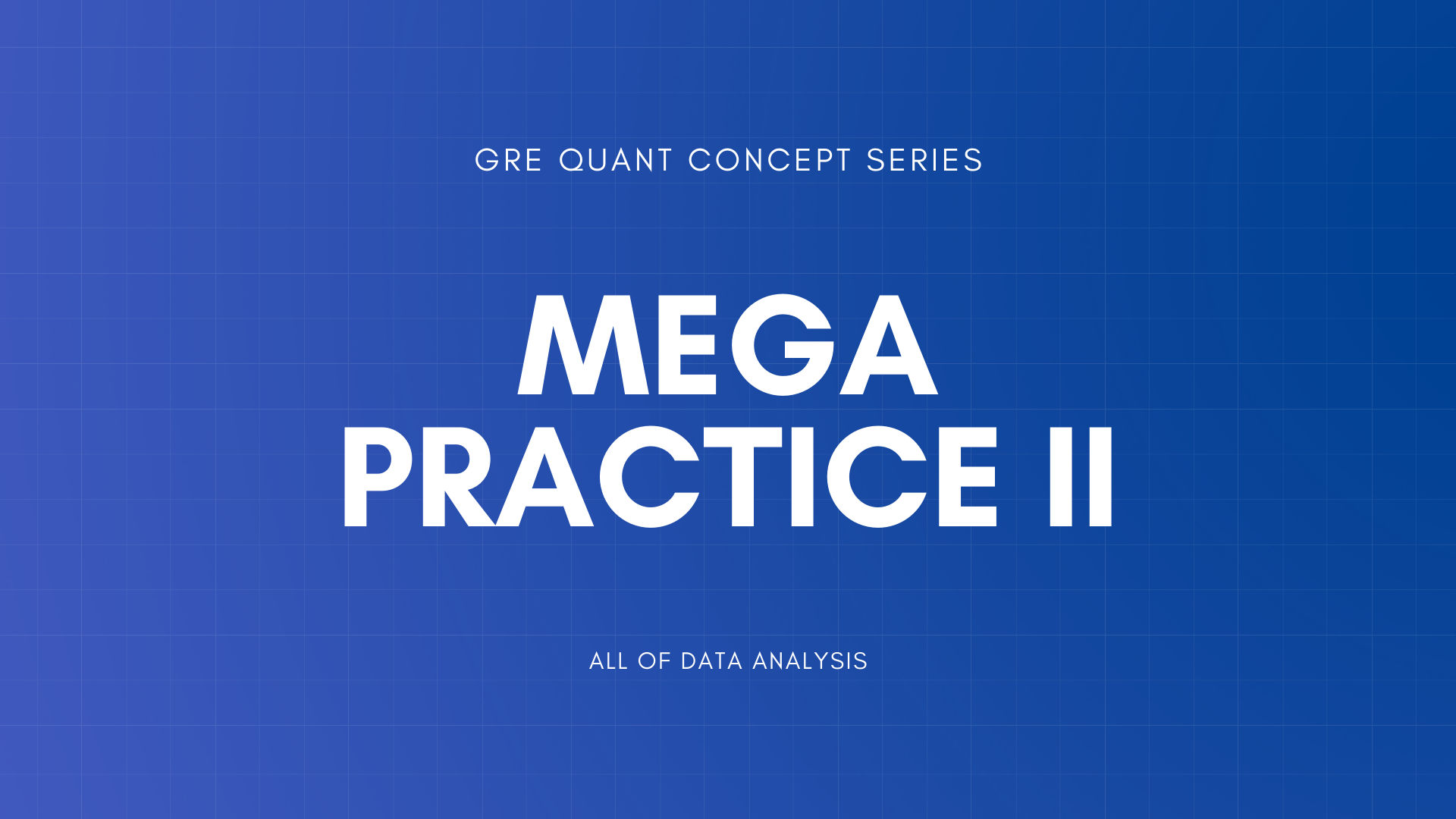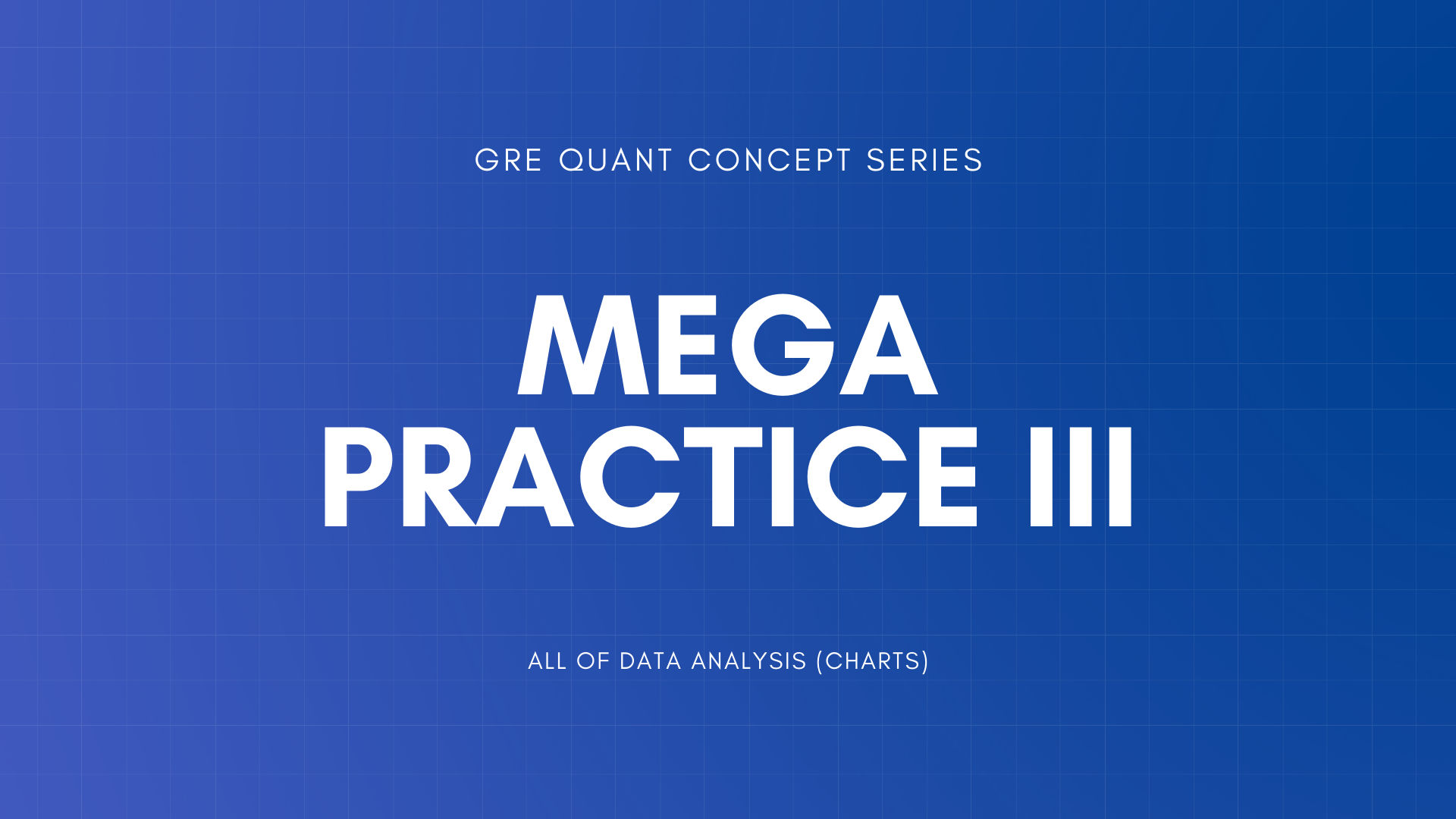GRE Quant Concept Series

Integers, Primes, Factors, and MultiplesFractions, Decimals, Percent, and Ratios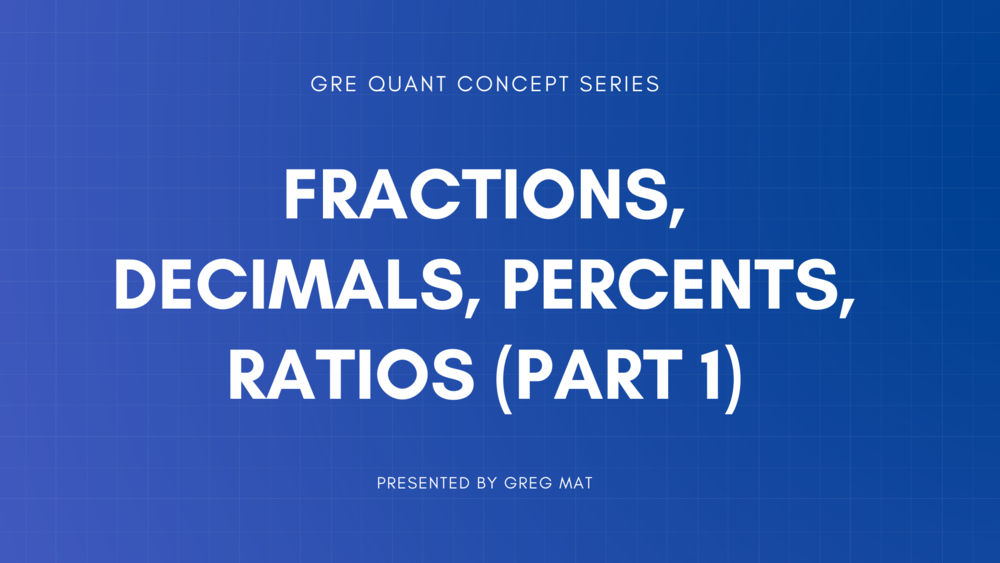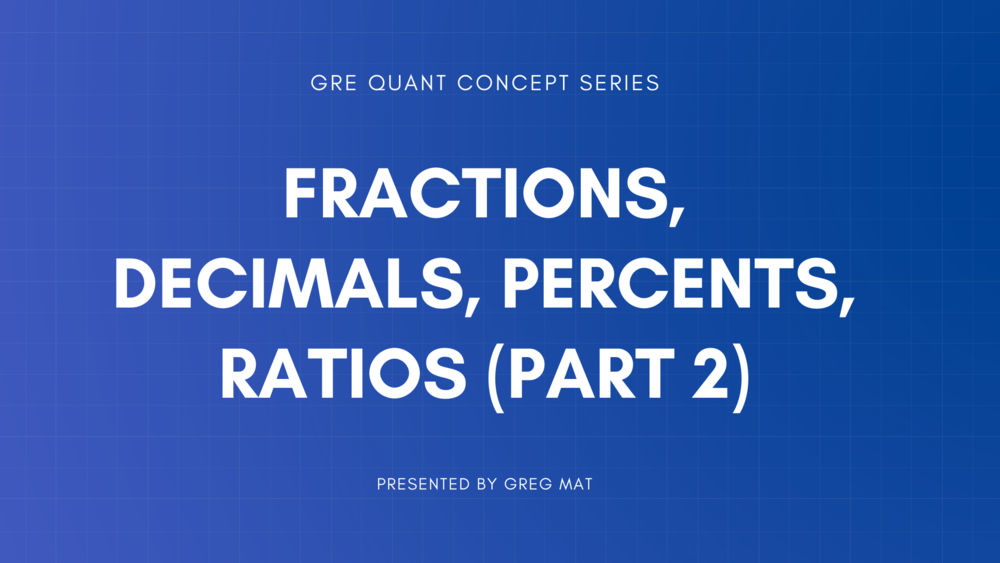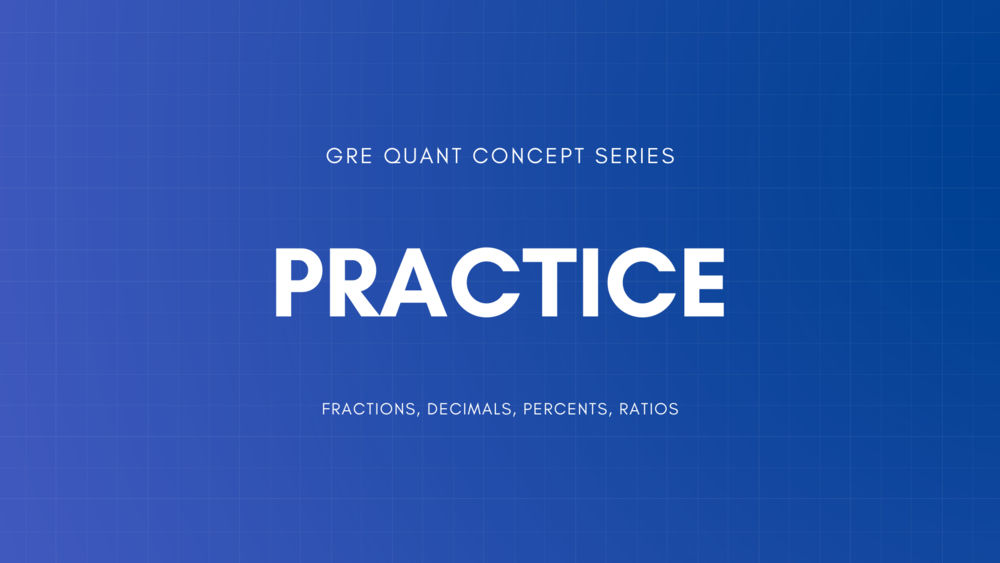Practice Incorporating All Previous Lessons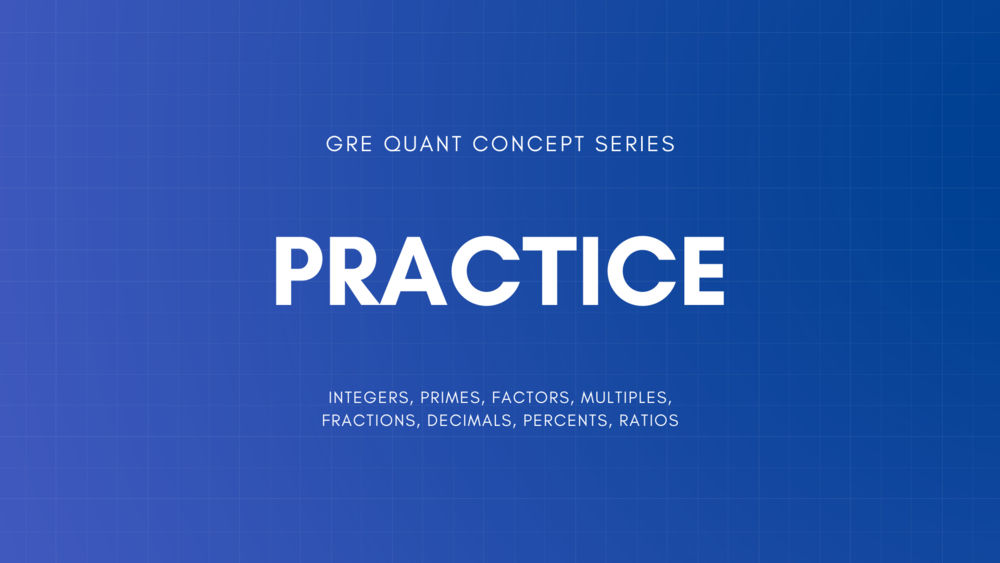Exponents and RootsPractice Incorporating All Previous Lessons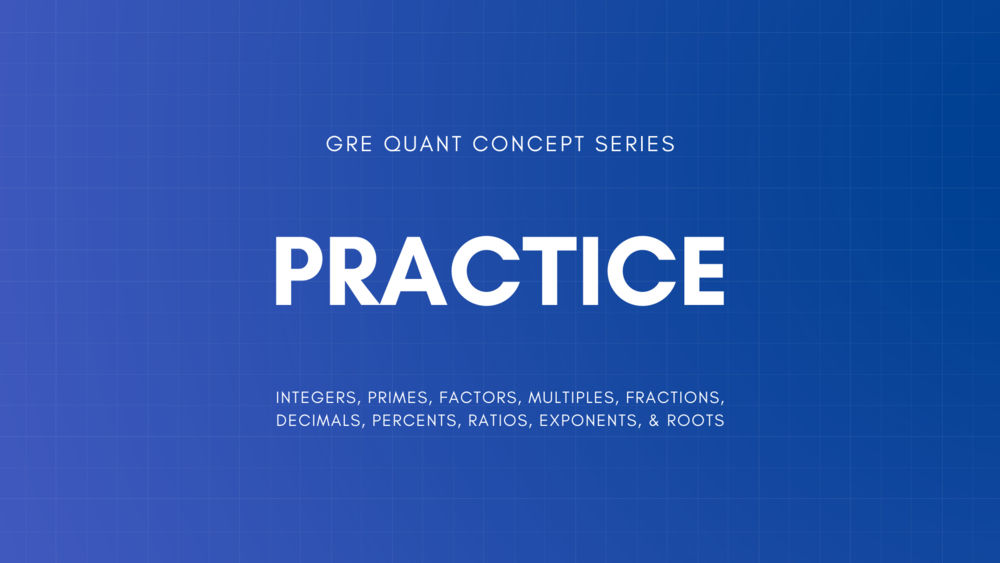Real Numbers and Absolute Value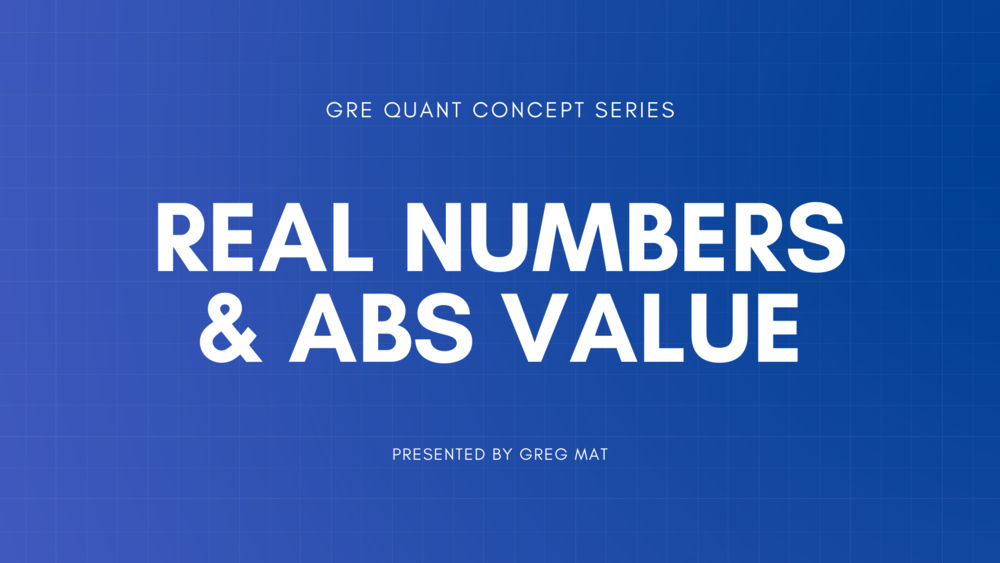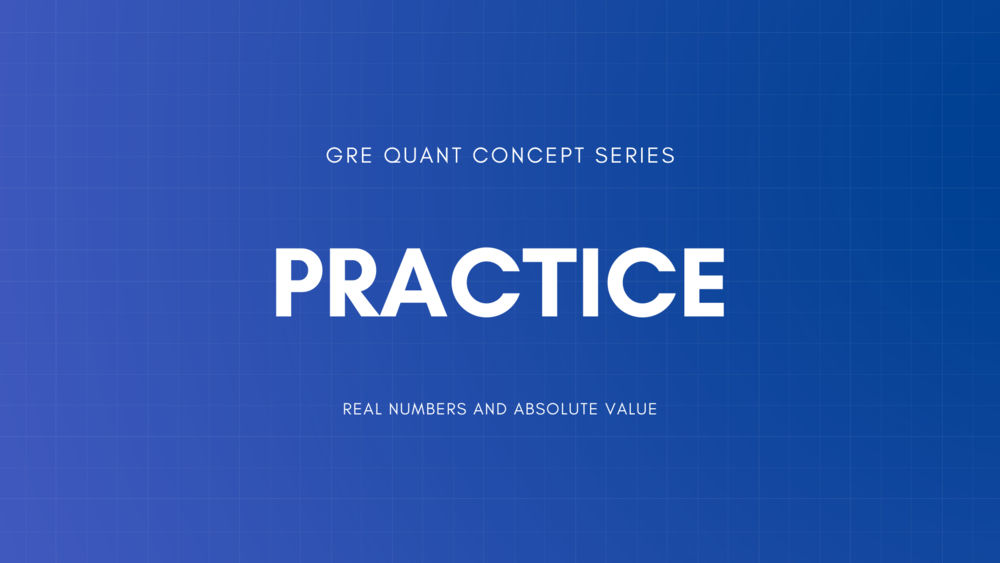Practice Incorporating All Previous Arithmetic LessonsAlgebraic ExpressionsExponent Rules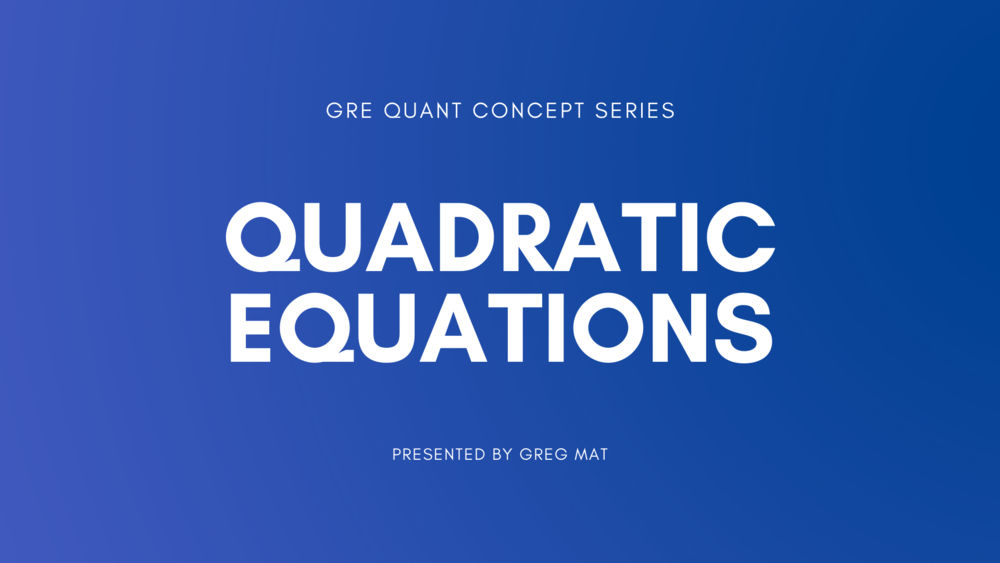Linear Inequalities and FunctionsApplications: Words to AlgebraWork and Distance Algebra ProblemsMixture and Interest ProblemsCoordinate Geometry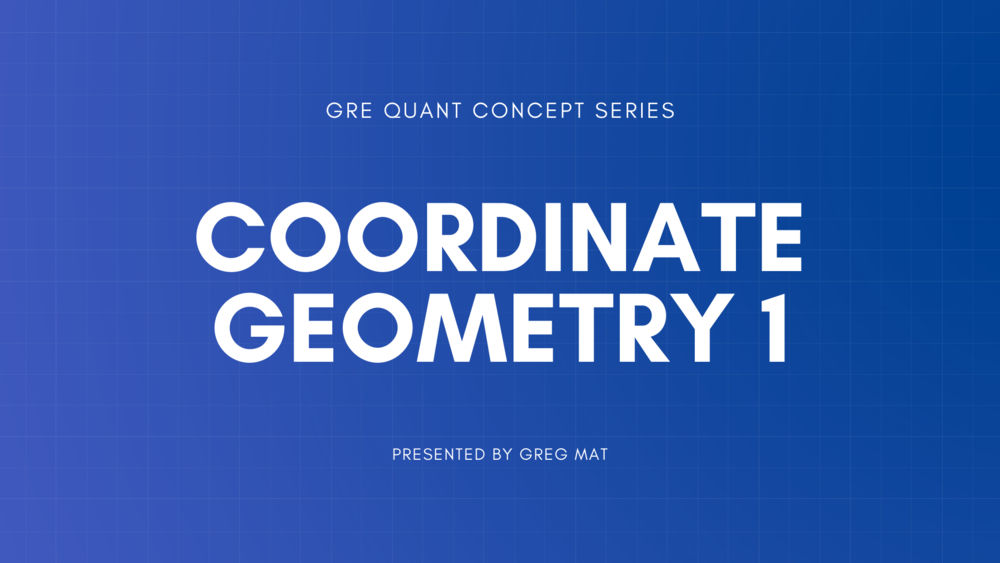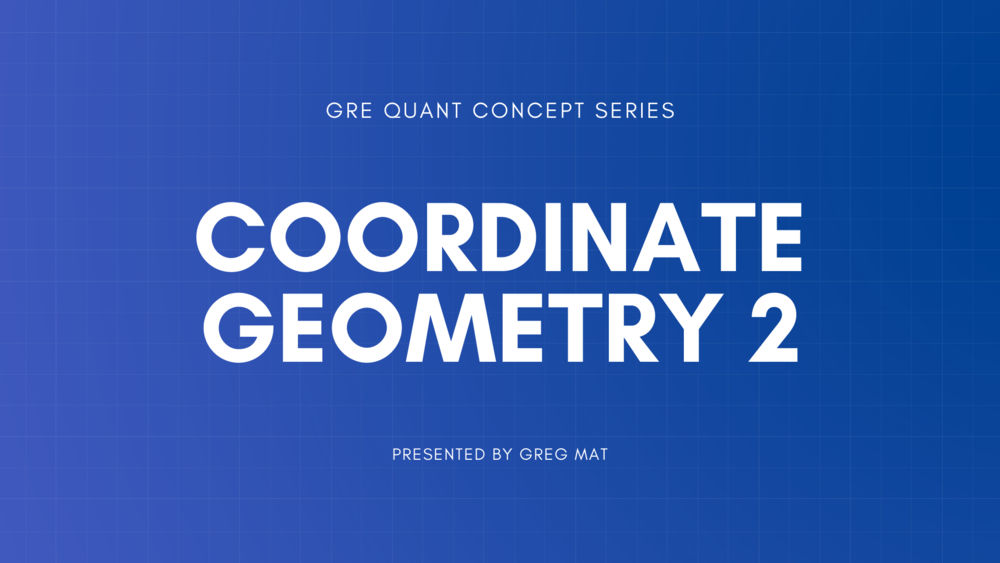Review of Algebra PracticeAngles, Lines, and PolygonsTrianglesCirclesPractice Incorporating All Previous Geometry Lessons3D Figures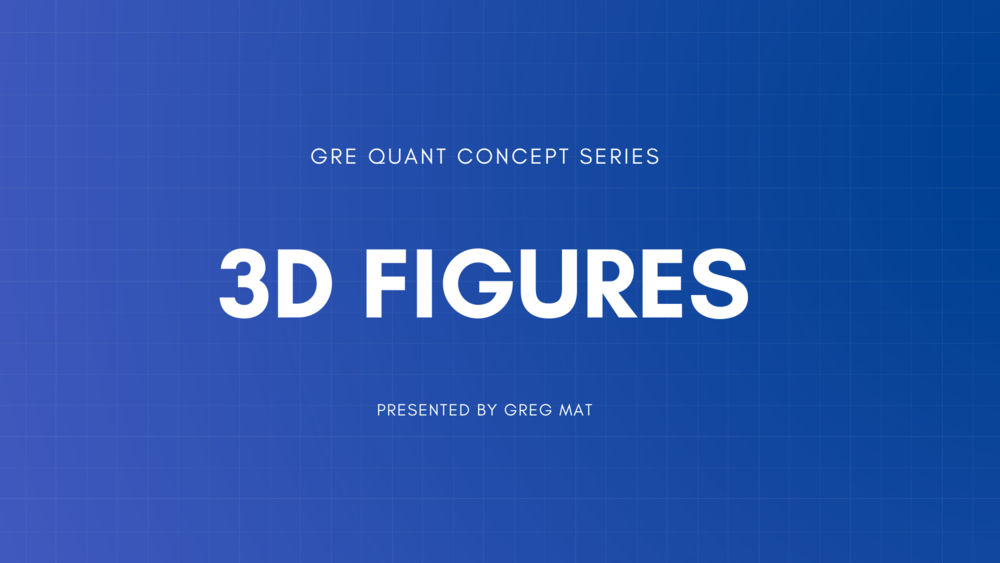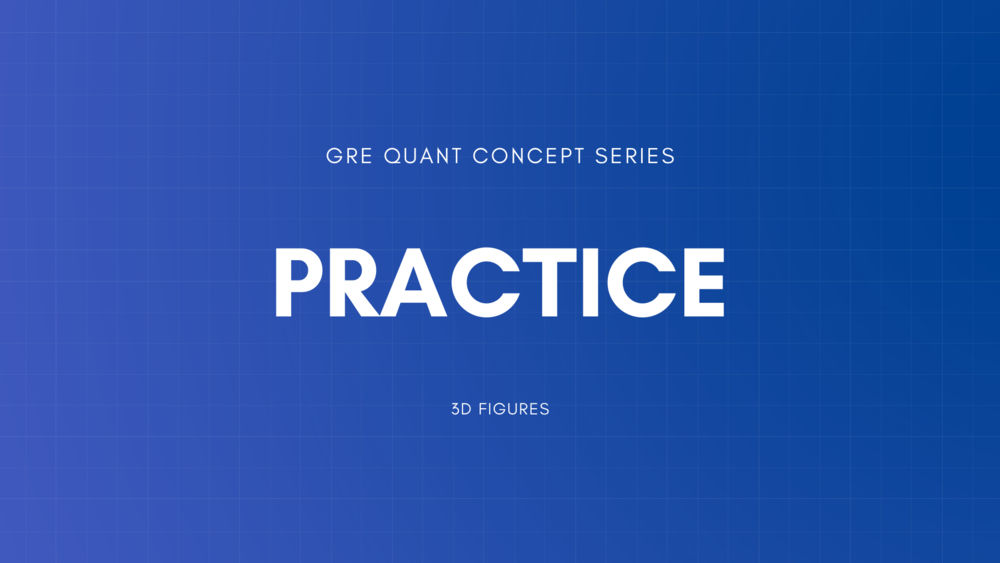Practice ALL Geometry ConceptsPresenting Data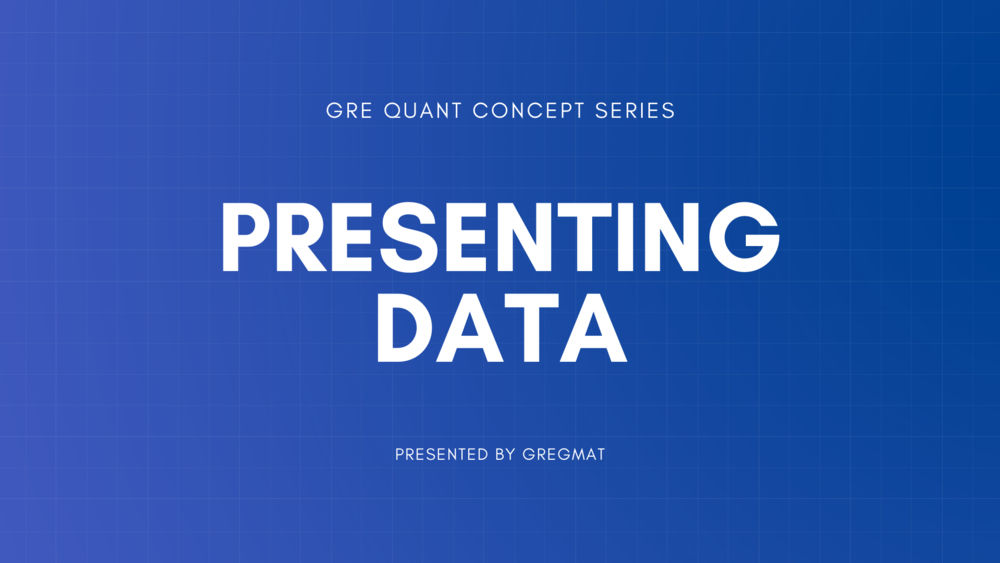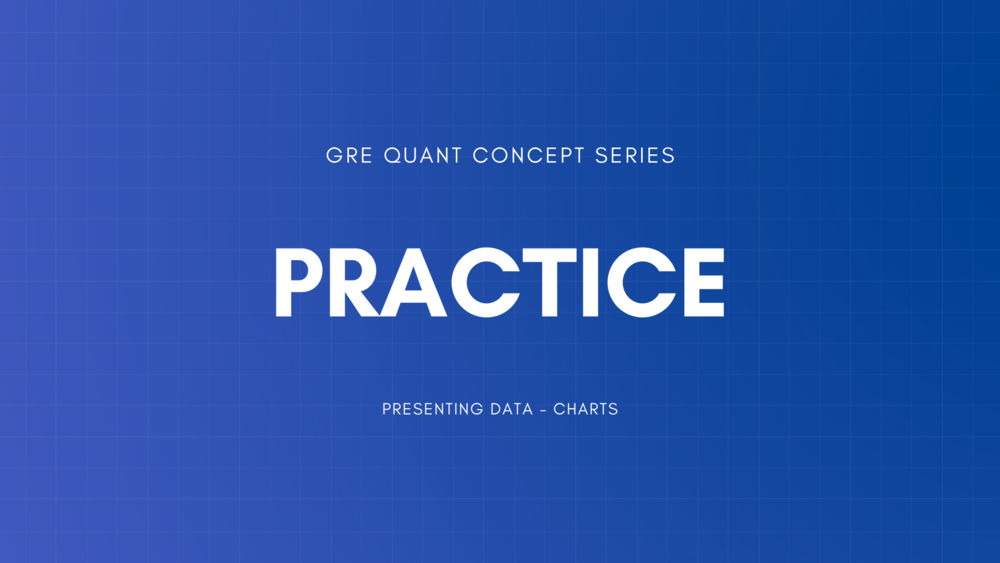Mean, Median, Mode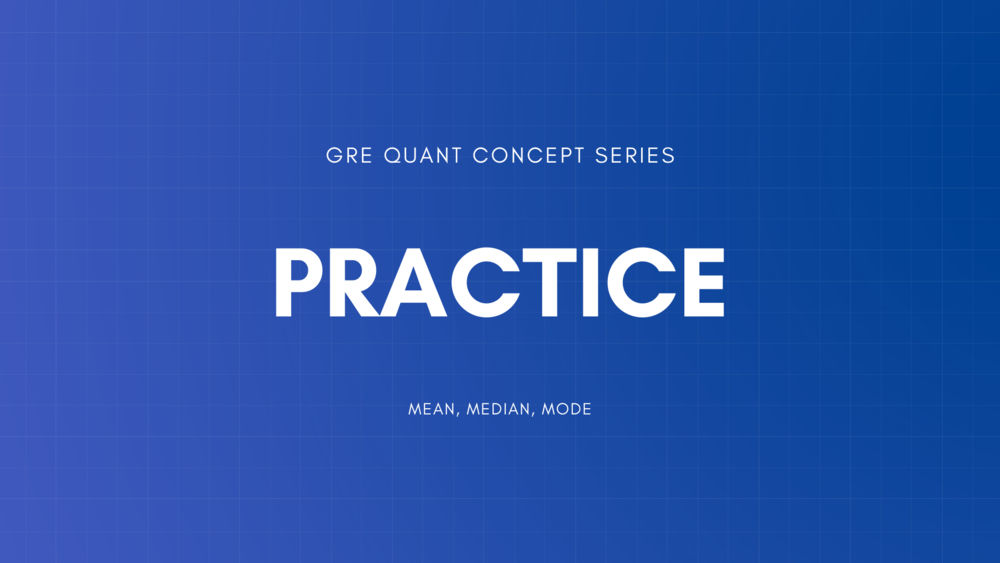Standard Deviation and Other ThingsOverlapping Sets and Multiplication Principle & Permutations Combinations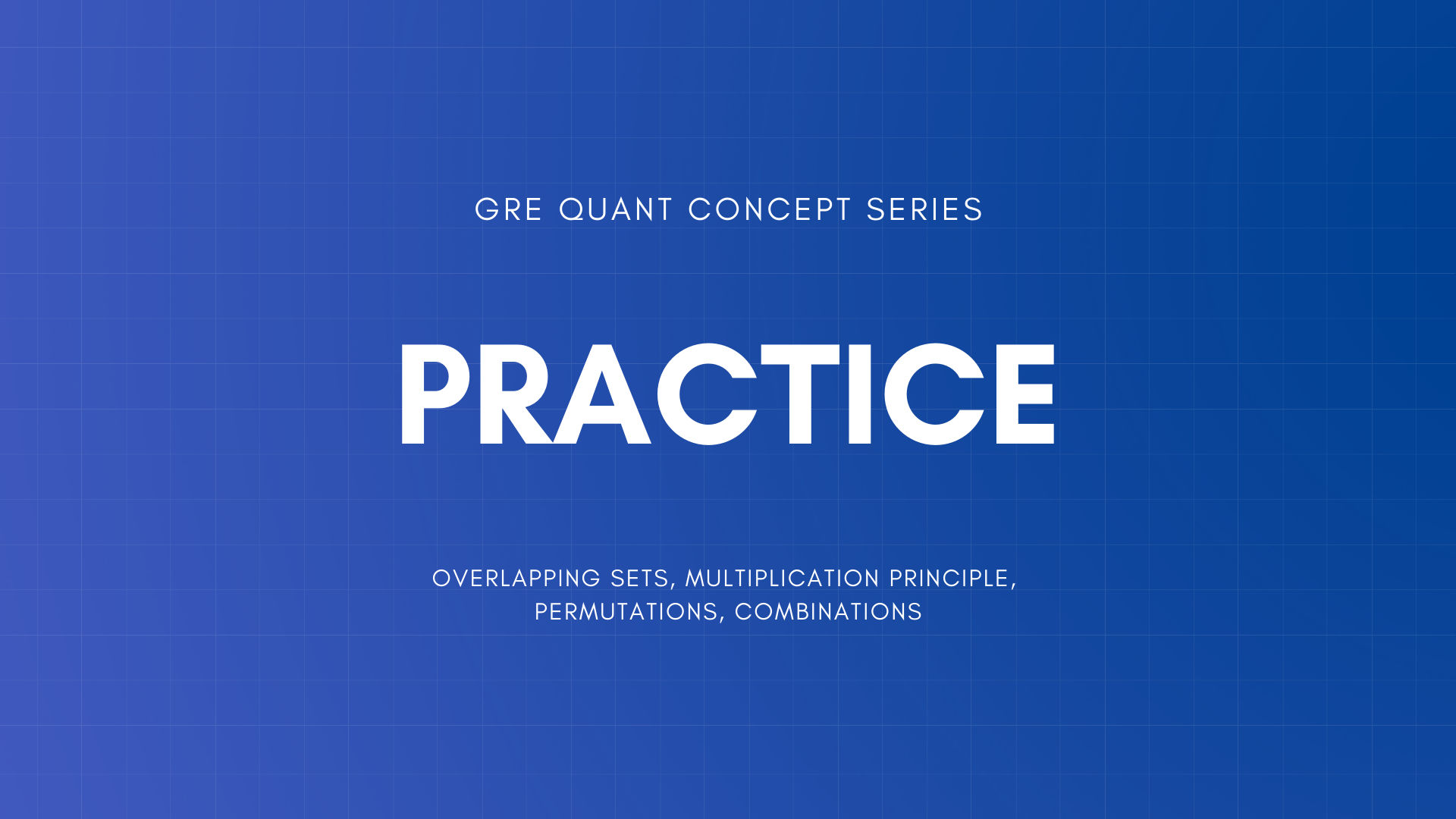Probability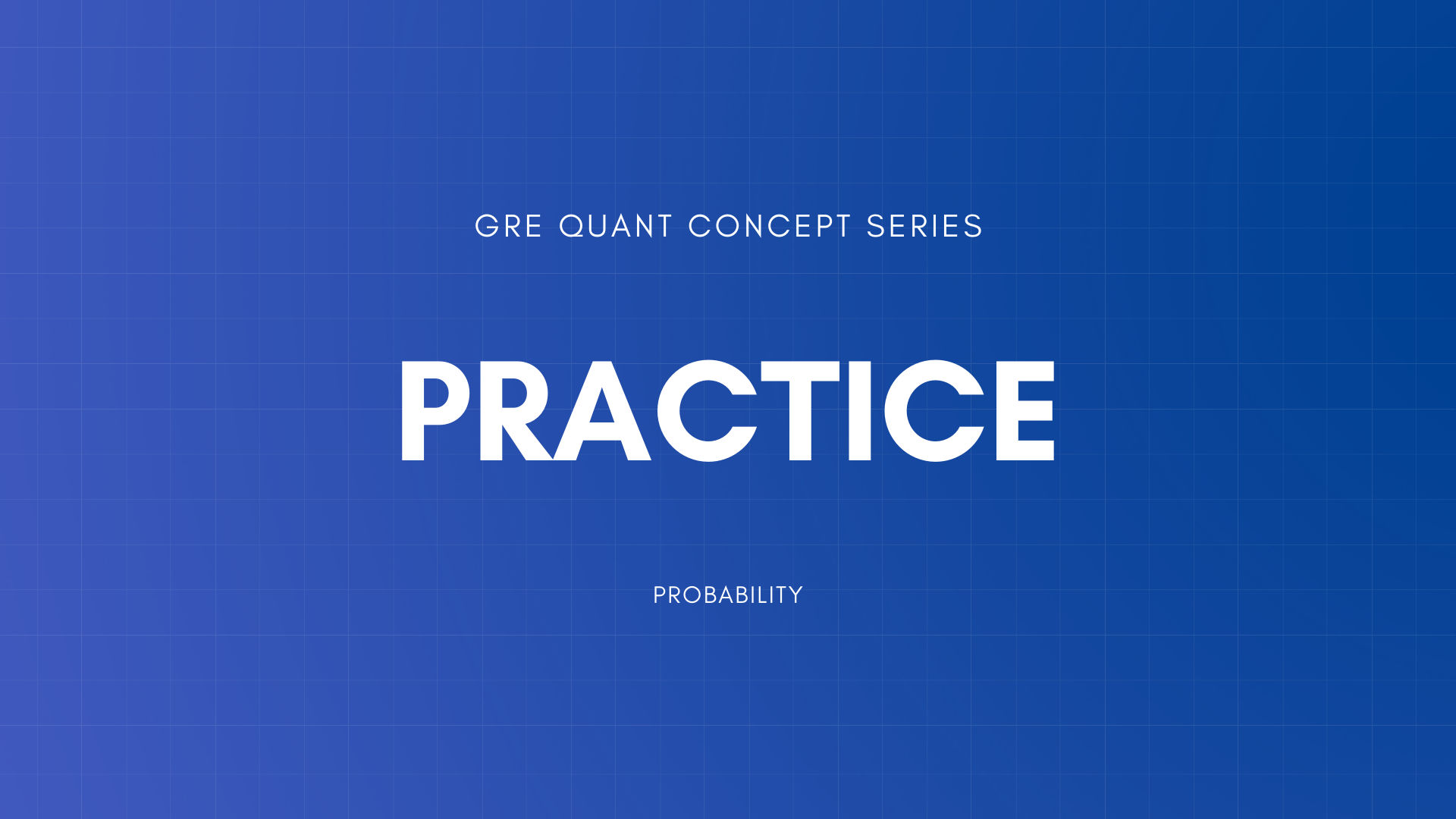Normal Distribution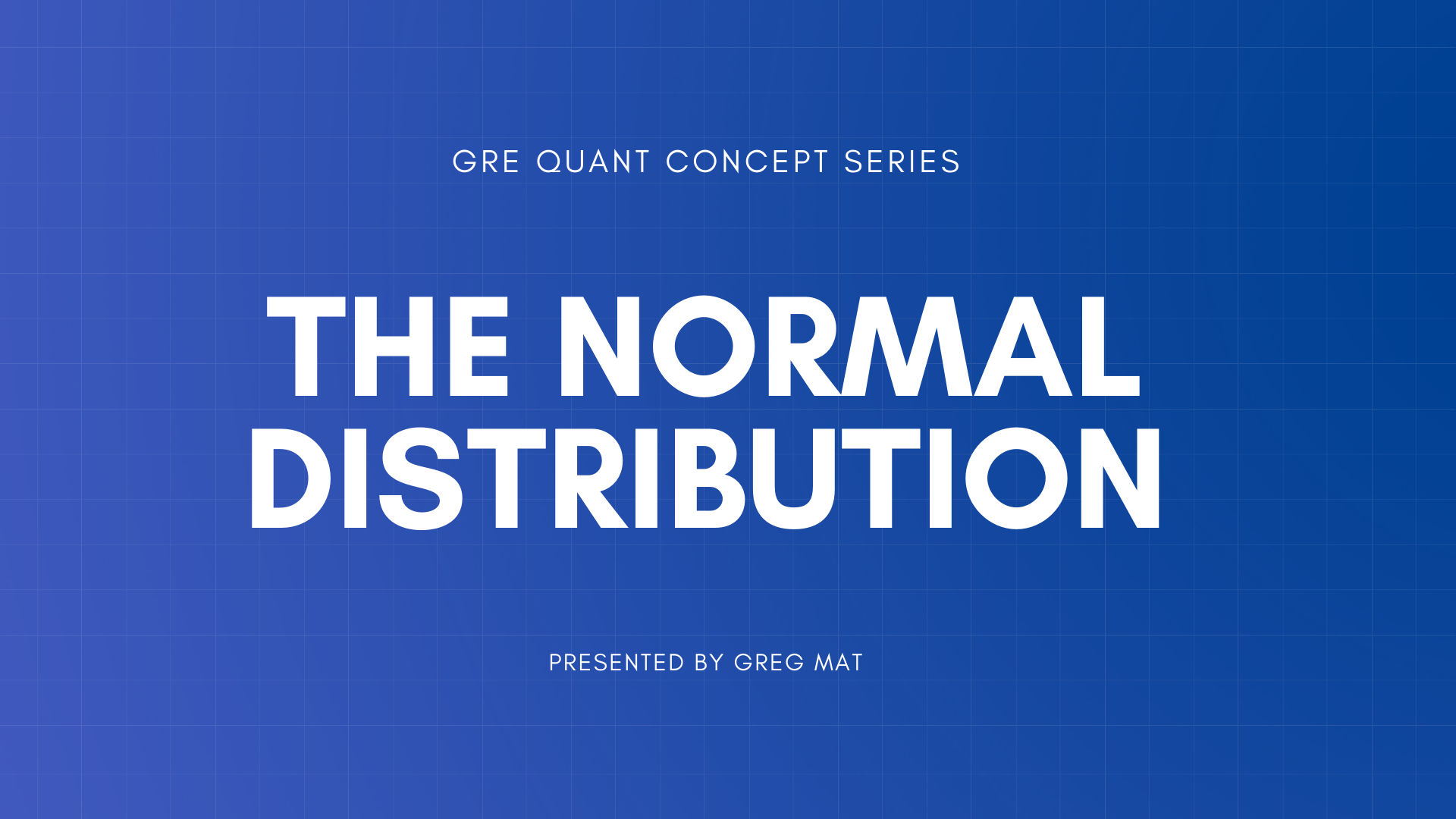Practice ALL Data Analysis Concepts